### IMO Shortlist 2005 problem G4

Kvaliteta:
Avg: 4.0
Težina:
Avg: 7.0
Let$ABCD$ be a fixed convex quadrilateral with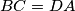$BC=DA$ and$BC$ not parallel with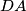$DA$. Let two variable points$E$ and$F$ lie of the sides$BC$ and$DA$, respectively and satisfy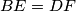$BE=DF$. The lines$AC$ and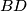$BD$ meet at$P$, the lines$BD$ and$EF$ meet at$Q$, the lines$EF$ and$AC$ meet at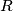$R$.

Prove that the circumcircles of the triangles$PQR$, as$E$ and$F$ vary, have a common point other than$P$.
Source: Međunarodna matematička olimpijada, shortlist 2005